# Calculating Derivatives and Derivative Rules in AP Calculus: Homework Help Resource Chapter Exam

Exam Instructions:

Choose your answers to the questions and click 'Next' to see the next set of questions. You can skip questions if you would like and come back to them later with the yellow "Go To First Skipped Question" button. When you have completed the practice exam, a green submit button will appear. Click it to see your results. Good luck!

### Page 1

#### Question 1 1. Which of the following statements is true, given g(t) below?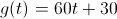#### Question 2 2. If the following graph is f(x), then which graph below could be f'(x)?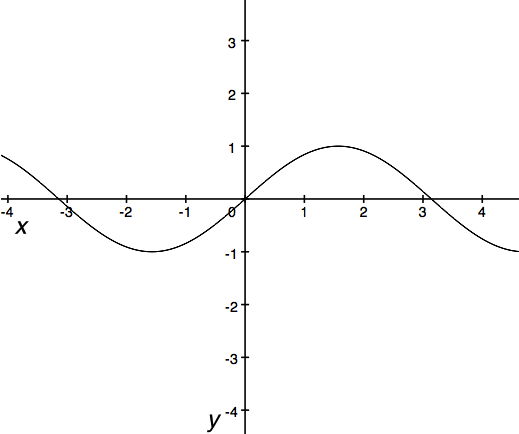#### Question 3 3. What is the highest-order derivative of f(x) that is NOT zero?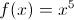#### Question 4 4. Find y'.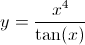#### Question 5 5. The derivative below may have been calculated from which function f(x)?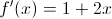### Page 2

#### Question 6 6. Evaluate the derivative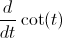#### Question 7 7. Find f'(x) for the following function.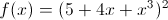#### Question 8 8. Which of the following statements is true about the derivative of f(x)?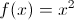#### Question 9 9. The graph x(t) represents the position of an object as a function of time. What is the acceleration of the object for t>0?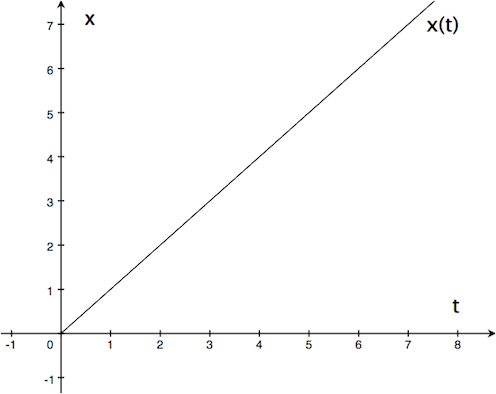#### Question 10 10. Differentiate f(x).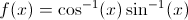### Page 3

#### Question 11 11. Differentiate g(x).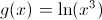#### Question 12 12. Find the jerk of the function shown below: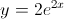#### Question 13 13. Find the derivative of f(x).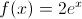#### Question 14 14. Find the derivative of f(x).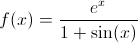#### Question 15 15. If h(t) is the height of an object as a function of time, then which of the following statements are true about h(t)? (Assume t>0.) A) When hitting the ground (at h(t)=0), the object's velocity is -2. B) When hitting the ground (at h(t)=0), the object's acceleration is -2. C) The object hits the ground at t=2.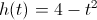### Page 4

#### Question 16 16. Find the derivative df/dt, given the function f(t).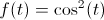#### Question 17 17. Find the derivative of f(x).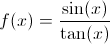#### Question 18 18. Find the derivative of f(x).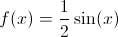#### Question 19 19. Find the derivative of f(x).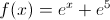#### Question 20 20. Find the second derivative of y(x).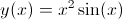### Page 5

#### Question 21 21. Find the derivative of f(x).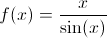#### Question 22 22. What is the derivative of f(x)?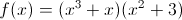#### Question 23 23. The function v(t) represents the velocity of an object as a function of time. Given the graph of v(t) below, which of the following statements is true?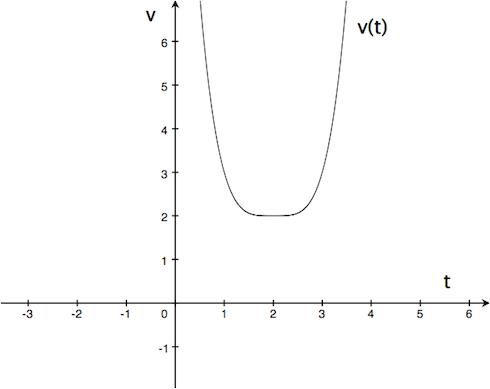#### Question 24 24. Find f'(t) given the function f(t):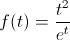#### Question 25 25. Find y'(x).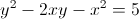### Page 6

#### Question 26 26. Differentiate the following function: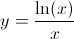#### Question 27 27. What is the derivative of y?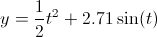#### Question 28 28. Find the derivative of f(x).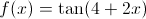#### Question 29 29. Find the second derivative of f(x) shown below: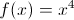#### Question 30 30. Evaluate.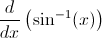#### Calculating Derivatives and Derivative Rules in AP Calculus: Homework Help Resource Chapter Exam Instructions

Choose your answers to the questions and click 'Next' to see the next set of questions. You can skip questions if you would like and come back to them later with the yellow "Go To First Skipped Question" button. When you have completed the practice exam, a green submit button will appear. Click it to see your results. Good luck!

Support